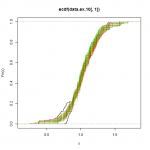September 14, 2017
By

Want to share your content on R-bloggers? click here if you have a blog, or here if you don't.In this set of exercises, we are going to explore some applications of probability functions and how to plot some density functions. The package `MASS` will be used in this set.

Note: We are going to use random numbers functions and random processes functions in R such as `runif`. A problem with these functions is that every time you run them you will obtain a different value. To make your results reproducible, you can specify the value of the seed using `set.seed(‘any number’)` before calling a random function. (If you are not familiar with seeds, think of them as the tracking number of your random number process.) For this set of exercises, we will use `set.seed(1)`. Don’t forget to specify it before every exercise that includes random numbers.

Answers to the exercises are available here. If you obtained a different (correct) answer than those listed on the solutions page, please feel free to post your answer as a comment on that page.

Exercise 1

Generating dice rolls Using the functions `runif` and `round`, simulate the results of 100 dice rolls.

Exercise 2

Let’s assume that we want to simulate a game in which we throw an unfair coin (success probability is 0.48) 10 times and you win \$10 every time the result is tails and lose \$10 when the result is heads. Simulate this game1000 time using `rbinom`, and find the expected amount of money you will gain or lose in this game using the simulated values.

Exercise 3

Simulate an experiment of throwing one dice 30 times using the function `rmultinom`, and find out how many 6’s are in the simulated sample.

Exercise 4

Obtain a vector that shows how many 1’s, 2’s,….6’s were obtained in the previous simulation.

Exercise 5

Simulate normal distribution values. Imagine a population in which the average height is 1.70 m with a standard deviation of 0.1. Use `rnorm` to simulate the height of 1000 people and save it in an object called `heights`.

a) Plot the density of the simulated values.
b) Generate 10000 values with the same parameters and plot the respective density function on top of the previous plot in red to differentiate it.

This plot will show you how much a sample with 10000 simulations approximate to the real normal distribution.

Exercise 6

Find the 90% interval of a population with mean = 1.70 and standard deviation = .1

Exercise 7

Simulate 100000 people with height (cm) and weight (kg) using the function `mvrnorm` with ` mu = c(170, 60) ` and
` Sigma = matrix(c(10,17,17,100), nrow = 2)`, and save it in an object called `population`.

Apply the function `summary` to `population` to get an idea of the values created.

Learn more about probability functions in the online course Statistics with R – Advanced Level. In this course you will learn how to

• work with different binomial and logistic regression techniques,
• know how to compare regression models and choose the right fit,
• and much more.

Exercise 8

Plotting bivariate distribution. Use the function `kde2d` to generate a two-dimensional kernel density of the matrix `population` and plot the values using `persp`.

Exercise 9

Simulating with a Bayesian approach. Unlike the frequentist statistics approach, Bayesian statistics assume the parameters of a distribution are a random variable with its own distribution. Let’s simulate a poisson variable.

a) Simulate a gamma variable with shape = 20 and scale = 0.5
b) Simulate using the previous value a poisson random variable

Exercise 10

Simulating one variable doesn’t make sense if you want to know the properties of a certain distribution. Repeat the previous simulation but create 100 poisson variables and plot the distribution.

R-bloggers.com offers daily e-mail updates about R news and tutorials about learning R and many other topics. Click here if you're looking to post or find an R/data-science job.
Want to share your content on R-bloggers? click here if you have a blog, or here if you don't.